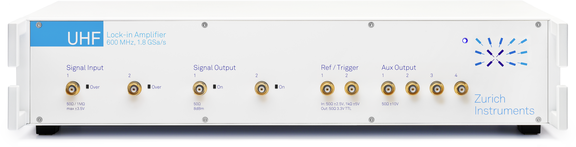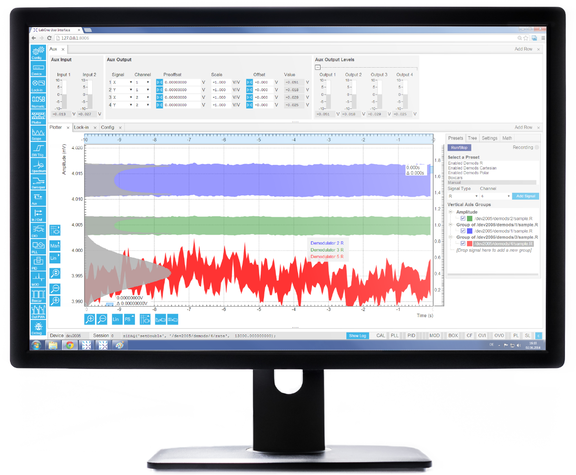# 锁相检测技术的原理

Principles of Lock-in Detection

## 简介Figure 1: 锁相放大器可测量待测信号相对于特定参考信号的幅值和 相位（甚至是完全淹没在噪声之中的信号）。

## 锁相放大器的工作原理

### 双相解调Figure 2: (a) 典型锁相测量过程的示意图。正弦信号一方面用来驱动 DUT，另一方面也作为参考信号使用。锁相放大器将对 DUT 的响应 进行分析，并输出相对于参考信号的信号幅值和相位。(b) 锁相放大原 理图：输入信号将分别与参考信号及其 90◦ 相移信号相乘。混频器输 出经过低通滤波后，将滤除噪声和 2ω 分量，并最终转化为极坐标。

\begin{align*} &R=\sqrt{X^2+Y^2},\\ &\Theta = atan2(Y,X) \end{align*}
(1)

Figure 2 (b)所示，为了以两个不同相位解调输入 信号，锁相放大器需要将输入信号进行拆分。相比于 模拟仪器，利用数字技术拆分信号可以避免任何 SNR 损失和通道间失配问题。

### 时域中的信号混频

$cos(x)=\frac{1}{2}e^{+ix}+\frac{1}{2}e^{-ix}$
(2)

\begin{align*} V_S(t)&=\sqrt{2}R\cdot cos(\omega_St+\Theta) \\ &= \frac{R}{\sqrt{2}}e^{+i(\omega_st+\Theta)}+\frac{R}{\sqrt{2}}e^{-i(\omega_st+\Theta)}. \end{align*}
(3)

$V_r(t)=\sqrt{2}e^{-i\omega_rt}=\sqrt{2}cos(\omega_rt)-i\sqrt{2}sin(\omega_rt).$
(4)Figure 3: 在复平面中表示的解调过程。(a) 输入信号 Vs(t) 可表示为 两个反向旋转的矢量之和。(b) 矢量在实轴 x 轴上的投影彼此叠加，而 在虚轴 y 轴上的投影则相互抵消。(c) 在旋转坐标系中，逆时针方向 矢量保持静止，顺时针方向矢量以观察者两倍的角速度旋转。请注意， 依照惯例，如果逆时针方向矢量在参考信号之前，则 ϴ 为正值。

\begin{align*} Z(t) &= X(t)+iY(t)=V_S(t)\cdot V_r(t)\\ &= R\left [ e^{i\left [ (\omega_s-\omega_r)t+\Theta \right ]} +e^{-i\left [ (\omega_s+\omega_r)t+\Theta \right ]}\right ], \end{align*}
(5)

$Z(t)=R\cdot e^{i[(\omega_s-\omega_r)t+\Theta]}.$

(6)

$Z(t)=R\cdot e^{i\Theta}.$
(7)

Equation 7 代表解调后的信号和锁相放大器主要输 出：绝对值 |Z| = R 为信号的均方根幅值，参数 arg(Z) = ϴ 为输入信号相对于参考信号的相位。Figure 4: (a) 峰值为 0.5 V 的输入信号 Vs （红色）与同频参考信号 Vr（蓝色）相乘。(b) 产生的信号将带有 Vs 和 Vr 频率的频率分量。直 流偏移量为 0.17 V，是输入信号的同相分量 X。(c) 输入信号 Vs 与非 同频参考信号 Vr 相乘。(d) 产生的信号将带有一个直流偏移量和两倍 于 fs − fr 和 fs + fr 频率的频率分量。平均信号始终为零。

\begin{align*} X&= Re(Z)=\left \langle V_s(t)cos(\omega_st) \right \rangle=R \; cos \; \Theta,\\ Y&= Im(Z)=-\left \langle V_s(t)sin(\omega_st) \right \rangle=R \; sin \; \Theta. \end{align*}
(8)

Figure 4为示波器显示的混频和滤波前后的不同信 号。 Figure 4 所示为一段时间的正弦信号示例 Vs 和 Vr，两信号频率相同，分别为 ωs 和 ωr。Figure 4 (b) 中的蓝线为混频后信号，主要为 2ω 分量。滤波后（绿 线），仅保留直流分量，其值等于 Vs 的同相幅值 X。 如Figure 4 (c),) 所示，如果信号频率与参考频率不同， 则混频后的信号不再是简单的正弦波，并且滤波后的 平均值始终为零，如Figure 4 (d)所示。这是一个典 型的同步检测示例，检测中仅提取出与参考频率具有 相干关系的信号，滤除了所有其他信号。

### 频域内的信号混频

Figure 5 (a) 所示为时域中的有噪正弦信号，经过傅里 叶变换后，在频域中如  Figure 5 (b)所示。正弦信号在 频谱中 +fs 和 −fs 频率处出现峰值。零频率处的较小 峰值是由输入信号的直流偏移所导致的。 Figure 5 (c) 中的蓝线表示混频后的时域信号。 Figure 5 (d) 所示 的关联频谱与图 (b) 中基本一致，但向低频方向平移 了相当于 fr 参考频率的距离。Figure 5: 解调前后信号时域图与频域图之间的关系。(a) 一段时间内 叠加了噪声的正弦输入信号。(b) (a) 图信号的频域图。(c) 与参考信 号混频（蓝线）并经过低通滤波（红线）后，fBW 内的信号频谱保留。 (d) 在频域中，混频过程将频率分量平移 −fr。随后，滤波器以零点为 中心提取宽度为 fBW 的狭窄频带。请注意 −fs 频率分量，它来自输入 信号中的偏移和 1/f 噪声。要获得准确的测量值，必须通过适当的滤 波来抑制此分量。

### 频域中的低通滤波

$Q_{out}(\omega)=H(\omega)Q_{in}(\omega).$
(9)

H(ω) 称为滤波器传递函数。Qin(ω) 与 Qout(ω) 分别 是时域中输入信号 Qin(t) 和输出信号 Qout(t) 的傅里叶变换。Figure 6: (a) 一阶 RC 滤波器及其传递函数公式。(b) 通过将多个 RC 滤波器级联可以针对更高频率获得更陡的滚降特性。最终的传递函数 为各滤波器传递函数相乘。

$H(\omega)=\frac{1}{1+i\omega\tau},$
(10)Figure 7: 中的蓝线为 RC 滤波器传递函数 H(ω) 的波特图。 同时，还绘制了具有相同滤波时间常数 τ 的高阶滤波器 (n = 2, 4, 8) 的传递函数，从图中可以看出，高阶滤波器的信号带宽 f−3dB 相对要 低得多。(c) 时域中对应的阶跃响应函数。通过级联多个滤波器，可以 显著增加达到同一精度的稳定时间。这与相位延迟的增大（从 (b) 中 可以看出）有关。级联 RC 或积分滤波器的另一个优点是，不会出现 巴特沃斯滤波器等类型滤波器中存在的时域内超调问题。Figure 8: 相同的一组图表，但 Figure 8 中所有滤波器的 截止点 f−3dB 相同，而时间常数不同，分别为 τ = 0.16、0.10、0.069、 0.048。(a) 高阶滤波器针对更高频率的滚降更陡。(b) 高阶滤波器的 相位延迟更大，会对反馈应用造成不良影响。(c) 时域内的阶跃响应函 数，以一阶滤波器时间常数 τ 1 为单位。尽管低阶滤波器在初始时对 输入信号变化的响应更为迅速，但这个优势会随时间的推移而逐渐减 小，在某一时间点，高阶滤波器甚至会超越低阶滤波器，如插图所示。

 阶数 时间 滚降系数 带宽，单位：1/τ 稳定时间，单位：τ n 常数 τ dB/oct dB/dec f-3dB fNEP fNEP/f-3dB 63.2% 90% 99% 99.9% 1 1 6 20 0.159 0.250 1.57 1.00 2.30 4.61 6.91 2 1 12 40 0.102 0.125 1.23 2.15 3.89 6.64 9.23 3 1 18 60 0.081 0.094 1.16 3.26 5.32 8.41 11.23 4 1 24 80 0.069 0.078 1.13 4.35 6.68 10.05 13.06 5 1 30 100 0.061 0.069 1.12 5.43 7.99 11.60 14.79 6 1 36 120 0.056 0.062 1.11 6.51 9.27 13.11 16.45 7 1 42 140 0.051 0.057 1.11 7.58 10.53 14.57 18.06 8 1 48 160 0.048 0.053 1.10 8.64 11.77 16.00 19.62
Table 1: 具有相同时间常数的 n 阶 RC 滤波器的滤波特性概况。在动态应用中，通常需要考量 f−3dB 和稳定时间，而在噪声测量中，保证 fNEP 正 确则是获得准确结果的关键。通过上述关系，针对带宽相同而阶数不同的滤波器，可以轻松算出滤波时间常数。

$H_n(\omega)=H_1(\omega)^n=(\frac{1}{1+i\omega\tau})^n.$
(11)

Figure 8 (a) and (b) 所示为不同阶数滤波器的波特图， 这些滤波器带宽同为 f−3dB，但时间常数不同。 Table 1 中提供了相应滤波特性之间的数字关系。

$Z(\omega)=V_s(\omega-\omega_r)H_n(\omega).$
(12)

### 时域中的低通滤波器

Table 1 列出了时间常数 τ 相同、阶数不同的各滤波 器达到最终值的 63.2%、90%、99% 和 99.9% 所需 的时间。假设采用 1 MHz 信号，并使用带宽为 1 kHz 的 4 阶滤波器在 1 MHz 周围进行滤波。通过 Table 1 中列出的数值，可以推出时间常数为 69 μs，达到 1% 误差所需的稳定时间为 0.7 ms。

## 信号动态特性和解调带宽

$V_s(t)=\left [ 1+h\, cos(\omega_mt) \right ]\,cos(\omega_ct+\varphi_c)$
(13)Figure 9: 调幅信号：绿线表示载波输入信号（为便于说明，以较低频 率显示）。蓝线表示信号幅值，为输入信号包络。Figure 10: (a) 旋转参考坐标系内的调幅信号是一个长度与时间相关 的矢量。瞬时信号由蓝色粗箭头表示；较窄的箭头表示 AM 信号的两 个边带。(b) 和 (c) 展示了解调输入信号的正交分量和同相分量：蓝线 为未经过滤波的信号，黑色虚线、红线和青色线分别表示以 f−3dB = 500 Hz、100 Hz 和 20 Hz 的滤波器进行滤波后的信号。(d) 以带宽 不同的三种滤波器进行滤波后的解调信号频谱（黑色、红色和青色曲 线）。

• 幅值 R(t) = 1 + h cos(ωmt) 的时间相关性
• 幅值 ⟨R(t)⟩ 的平均值
• 调制指数 hFigure 11: (a) 含噪声的输入信号将生成含噪声的解调信号（蓝线）。 无噪声的基底信号如图中黑色虚线所示。(b) 使用带宽 f−3dB = fm = 100 Hz 的滤波器将滤除大部分噪声，但也会影响被测信号。(c) 与 (b) 类似，但带宽 f−3dB = fm/5 = 20 Hz。

## 实现高信噪比Figure 12: 典型实验的噪声频谱定性分析。测量频率应从背景噪声较 小的区域中选择，避免技术性噪声源引入的离散峰值。在本例中，由 于低频时 f2 位于 1/f 噪声上方只有白噪声的区域，采用相同滤波器带 宽时，获得的结果要优于 f1

Figure 12 中的频谱定量分析展示了不同噪声源具有 不同的频率相关性：对于所有实际频率，约翰逊噪声 的频谱比较平坦，因而构成了“白噪声”，而闪烁噪声 具有 1/f 的频率相关性（“粉红噪声”）。如果在选择调 制频率时具有一定的选择空间，则可以放大频谱中噪 声水平最低的部分。通常，频谱中包含白噪声特性的 更高频率效果更佳。 Figure 12 展示了这种方式：在 频率较低的 1/f 噪声区域，滤波器内的噪声（由蓝色 和灰色填充区域表示）更大。因此，由于噪声密度较 低，只要避免无线电和无线传输等其他噪声源，采用 相同滤波器带宽时，f2 对应的 SNR 高于 f1

$SNR=\frac{1\mu V}{127nV/\sqrt{Hz}\cdot\sqrt{f_{NEP}}}=10$
(14)

## 发展现状Figure 13: (a) 模拟锁相放大器：信号分两条路径处理，在两条路径 中，都是先与参考信号混频，经滤波后再转换为数字信号。(b) 数字锁 相放大器：信号经数字化后与参考信号相乘并进行滤波。Figure 14: Zurich Instruments UHFLI 锁相放大器代表了最先进的锁相技术。此锁相放大器信号输入带宽为 600 MHz，解调带宽为 5 MHz，堪称 市场上迄今为止速度最快的锁相放大器。仪器宽 19 英寸，集成了大量功能（请参见 Figure 16），并采用最先进的仪器控制软件 LabOne® (请参 见) 进行控制。Figure 15: UHFLI 锁相放大器的 LabOne® 用户界面采用最新的 Web 浏览器技术。仪器能够通过多台计算机或平板电脑等设备上的多 个浏览器访问，并进行控制。每种信号分析和控制工具都有专用的选 项卡。部分功能以框图形式直观显示。Figure 16: Zurich Instruments UHFLI 的主要功能模块及相互之间的信号流框图。快速数字信号处理可以在时钟频率为 450 MHz 的 FPGA 内执 行，也可以在通过 USB 或 1GbE 端口连接且运行仪器控制软件 LabOne® 的计算机中执行。仪器的主要功能元件包括 8 个双相解调器、数字转换 器 (DIG) 和 FFT 功能的示波器 (Scope)、具有 PLL 功能的 PID 模块、运算单元 (AU)、带周期波形分析仪 (PWA) 的 Boxcar 平均器和脉冲计数器 模块 (CNT)。对于信号生成，仪器可提供正弦信号发生器 (OSC) 以及便于形成复杂信号形状的任意波形发生器 (AWG)。此外，在计算机上运行的 LabOne 控制软件还额外提供参数扫描仪、频谱分析仪、数值显示 (Num)、绘图仪、用于时域分析的软件触发器和谐波分析器 (Harm) 等功能。

 C. R. Cosens. A balance-detector for alternating-current bridges. Proceedings of the Physical Society, 46:818, 1934.
 W. C. Michels. A Double Tube Vacuum Tube Voltmeter. Rev. Sci. Instrum., 9:10, 1938.
 W. C. Michels and N. L. Curtis. A Pentode LockIn Amplifier of High Frequency Selectivity. Rev. Sci. Instrum., 12:444, 1941.
 Interview of Robert Dicke by Martin Hawrit.Niels Bohr Library and Archives, College Park, MD: American Institute of Physics, www.aip.org/history-programs/niels-bohr-library/oral-histories/4572, 1985. Accessed: 2016-10-21.
 Zurich Instruments HF2LI. http://www.zhinst.com/products/hf2li. Accessed: 2016-10-21.
 A. M. Skellett. The Coronaviser, an Instrument for Observing the Solar Corona in Full Sunlight. Proc Natl Acad Sci USA, 26(6):430, 1940.
 D. C. Tsui, H. L. Stormer, and A. C. Gossard. Two-dimensional magnetotransport in the extreme quantum limit. Phys. Rev. Lett., 48:1559, 1982.
 L. Gross et al. Bond-Order Discrimination by Atomic Force Microscopy. Science, 337(6100):1326, 2012.
 Stanford Research SR844. http://www.thinksrs.com/products/SR844.htm. Accessed: 2016-10-21.
 Wikipedia Article: Fourier Transform. https://en.wikipedia.org/wiki/Fourier_transform. Accessed: 2016-10-21.
 Wikipedia Article: Fourier Series. https://en.wikipedia.org/wiki/Fourier_series. Accessed: 2016-10-21.
 N. Thrane. Zoom-FFT. Brüel & Kjær Technical Review, (2):3, 1980.
 Zurich Instruments UHFLI. http://www.zhinst.com/products/uhfli. Accessed: 2016-10-21.